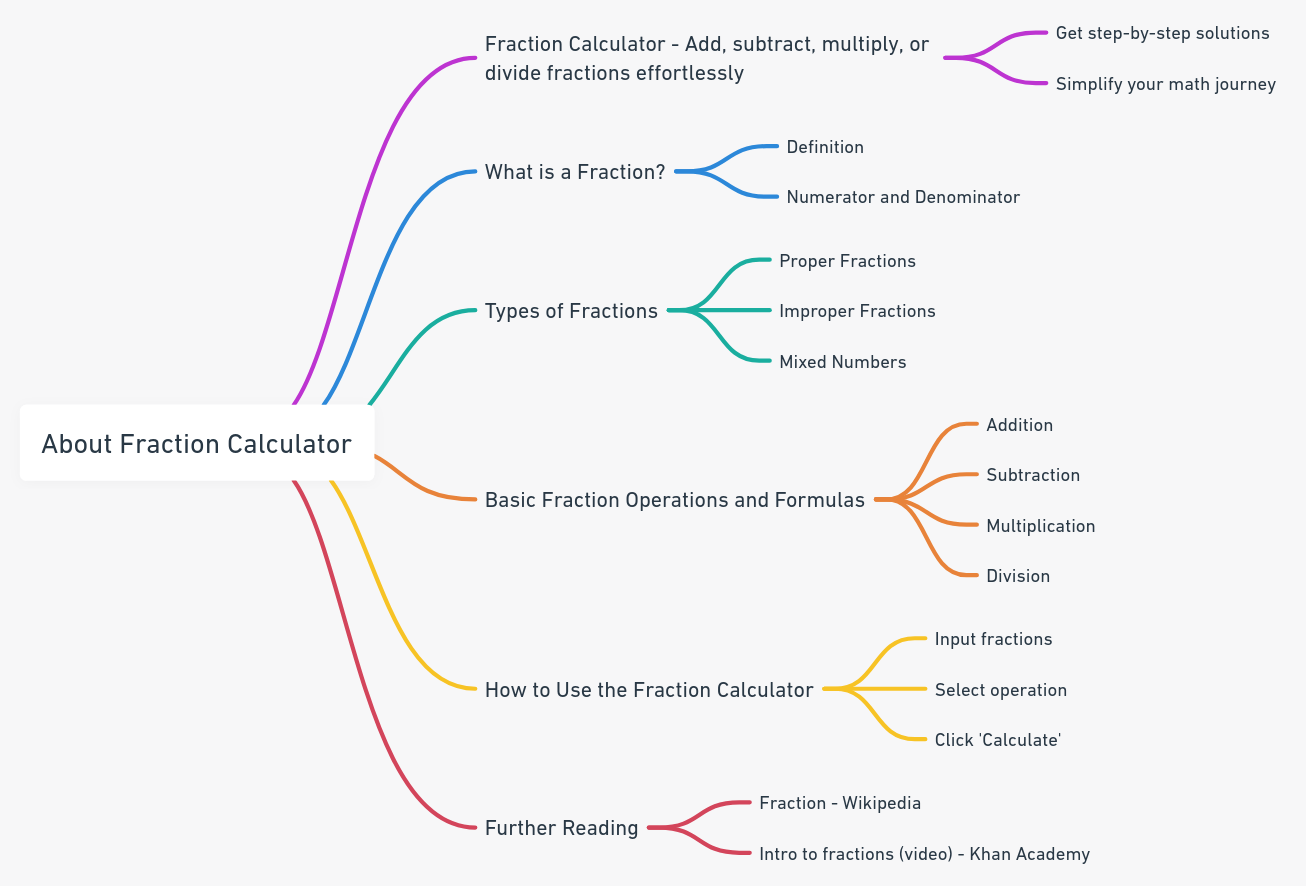Even The mini Tools Can Empower People to Do Great Things.

# Fraction Calculator: Step-by-Step Solutions for All Your Fraction Needs

Master fractions with our Fraction Calculator. Offering step-by-step solutions for addition, subtraction, multiplication, and division, this tool is your go-to guide for all your fraction needs.

Fraction Calculator
Enter the integer part of the mixed fraction (if it is a proper or improper fraction, you can leave it blank), the numerator, the denominator, select the operation symbol, then click the Calculate button.
 + − × ÷

Embed Fraction Calculator WidgetThe Fraction Calculator is used to calculate fractions addition, subtraction, multiplication and division (Step by Step).

## What is a Fraction?

A fraction is a mathematical expression that represents a part of a whole. It consists of two numbers: a numerator and a denominator. The numerator represents the number of parts you have, and the denominator indicates the total number of equal parts that make up the whole.

### Types of Fractions

1. Proper Fractions:The numerator is smaller than the denominator (e.g., $$\frac{3}{4}$$).
2. Improper Fractions: The numerator is equal to or greater than the denominator (e.g., $$\frac{5}{4}$$ or $$\frac{4}{4}$$).
3. Mixed Numbers: A combination of a whole number and a proper fraction (e.g., $$1 \frac{1}{2}$$).

### Basic Fraction Operations and Formulas

1. Addition: $$\frac{a}{b} + \frac{c}{d} = \frac{ad + bc}{bd}$$
2. Subtraction: $$\frac{a}{b} - \frac{c}{d} = \frac{ad - bc}{bd}$$
3. Multiplication: $$\frac{a}{b} \times \frac{c}{d} = \frac{ac}{bd}$$
4. Division: $$\frac{a}{b} \div \frac{c}{d} = \frac{ad}{bc}$$

## How to Use the Fraction Calculator

Our Fraction Calculator is designed to provide you with accurate and quick solutions for adding, subtracting, multiplying, and dividing fractions. To use the calculator, input proper or improper fractions in the designated fields, select the desired math operation, and click "Calculate." The calculator will provide the answer in lowest terms or as a mixed number.

## Reference:

Reference this content, page, or tool as:

"Fraction Calculator" at https://miniwebtool.com/fraction-calculator/ from miniwebtool, https://miniwebtool.com/

by miniwebtool team. Updated: Oct 30, 2023